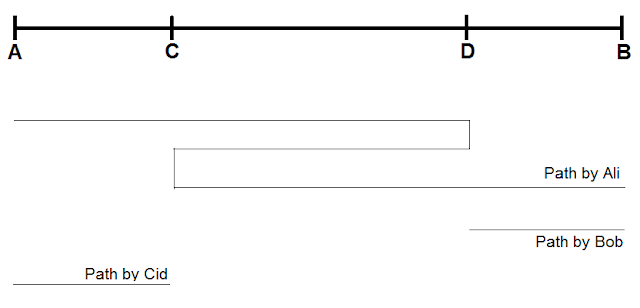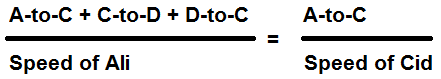### Solution 5 & 6

Solution 5

The distance between Town Y and Town Z is 7 km .
At the beginning , both Ali and Bob are at Town Y .
Ali walked from Town Y to Town Z at a constant speed .
After Ali had walked for 1 km , Bob discovered that Ali had left his belongs at Town Y .
Bob immediately ran after Ali at a constant speed of 4 km/h .
After handing over the belongings to Ali , Bob turned back and started running towards Town Y at the same speed .
Given that both Ali and Bob reached Town Z and Y respectively at the same time , what was Ali's speed ?

(Draws a line representing distance between Y , Z and X*)Since both Bob and Ali reached Y and Z respectively at the same time ,

Time taken for Bob from Y to X =
Time taken for Bob from X to Y =
Time taken for Ali from 1 km point to X =
Time taken for Ali from X to Z

(All the time taken are the same*)

Since time taken for Ali from 1 km point to X = Time taken for Ali from X to Z ,
we can conclude that x km = y km = (7-1) / 2 = 3 km

Bob from X to Y :
Distance = Speed x Time
( 3 + 1 ) km = 4 km/h x Time
Time = 1 hour

Ali from X to Z :
Distance = Speed x Time
3 km = Speed x 1 hour
Speed = 3 km/h

Ali's speed was 3 km/h .

Solution 6
Ali, Bob, Cid travel from Town Y to Town Z in a straight road .
They leave Town Y at the same time and travel at constant speed towards Town Z .
Ali drives a car at speed of 56 km/h .
Bob jogs at a speed of 8 km/h .
Cid walks at a speed of 7 km/h .
At the start , Ali gives a lift to Bob from Town Y and drops Bob somewhere along the road to Town Z before returning to pickn up Cid and travel to Town Z .
All three of them arrived at Town Z at the same time .
If Bob had jogged for 2.8km , how far did Cid walk ?

(This question can be confusing, so it's important to draw a line to see clearly distance traveled by each of the 3 persons*)(Ignore the vertical lines for Path by Ali*)
(D is the point where Ali drops Bob off and C is the point where Ali picks up Cid*)

Bob:
Distance = Speed x Time
2.8 km = 7 km/h x Time      (2.8 km is the distance from D to B)
Time = 0.4 hr

Time taken for Bob to travel from D to B = Time taken from Ali to travel from D to C to B

Ali:
Distance = Speed x Time
Distance = Speed x Time
D-to-C + C-to-D + D-to-B = 56 km/h x 0.4 hr
2 x ( C-to-D ) + 2.8 km = 22.4 km            ( D-to-B is 2.8 km and D-to-C is equal to C-to-D )
C-to-D = 9.8 km

Distance = Speed x Time
Time = Distance / Speed
Time taken for Ali to travel from A to C to D to C = Time taken for Cid to travel from A to C(A-to-C + 9.8 km + 9.8 km ) x (7) = (A-to-C) x (56)
7 x (A-to-C) + 137.2 = 56 x (A-to-C)
49 x (A-to-C) = 137.2
A-to-C = 2.8 km

Cid walked 2.8km .